# NCERT solutions for Mathematics Exemplar Class 6 chapter 6 - Mensuration [Latest edition]

#### Chapters## Chapter 6: Mensuration

Exercise
Exercise [Pages 93 - 102]

### NCERT solutions for Mathematics Exemplar Class 6 Chapter 6 Mensuration Exercise [Pages 93 - 102]

#### Choose the correct alternative:

Exercise | Q 1 | Page 93

Following figures are formed by joining six unit squares. Which figure has the smallest perimeter in figure?

•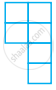•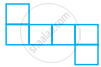•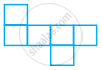•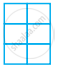Exercise | Q 2 | Page 93

A square shaped park ABCD of side 100m has two equal rectangular flower beds each of size 10m × 5m (Figure). Length of the boundary of the remaining park is ______.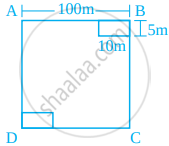• 360 m

• 400 m

• 340 m

• 460 m

Exercise | Q 3 | Page 93

The side of a square is 10 cm. How many times will the new perimeter become if the side of the square is doubled?

• 2 times

• 4 times

• 6 times

• 8 times

Exercise | Q 4 | Page 93

Length and breadth of a rectangular sheet of paper are 20 cm and 10 cm, respectively. A rectangular piece is cut from the sheet as shown in figure. Which of the following statements is correct for the remaining sheet?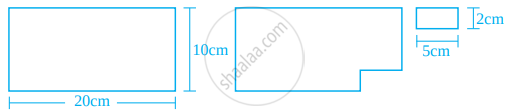• Perimeter remains same but area changes

• Area remains the same but perimeter changes

• Both area and perimeter are changing

• Both area and perimeter remain the same

Exercise | Q 5 | Page 94

Two regular Hexagons of perimeter 30 cm each are joined as shown in figure. The perimeter of the new figure is ______.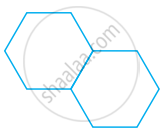• 65 cm

• 60 cm

• 55 cm

• 50 cm

Exercise | Q 6 | Page 94

In figure which of the following is a regular polygon? All have equal side except (i).

•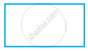•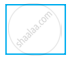•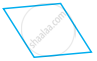•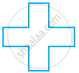#### Match the shapes (each sides measures 2cm) in column I with the corresponding perimeters in column II:

Exercise | Q 7 | Page 94
 Column I Column II (A)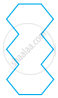(i) 16 cm (B)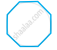(ii) 20 cm (C)(iii) 24 cm (D)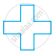(iv) 28 cm (v) 32 cm

#### Match the following:

Exercise | Q 8 | Page 95
 Shapes Perimeter (A)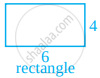(i) 10 (B)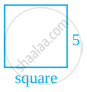(ii) 18 (C)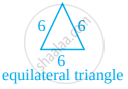(iii) 20 (D)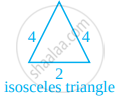(iv) 25

#### Fill in the blank:

Exercise | Q 9 | Page 95

Perimeter of the shaded portion in the given figure is ______.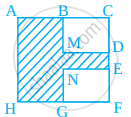AB + _ + _ + _ + _ + _ + _ + HA

Exercise | Q 10 | Page 95

The amount of region enclosed by a plane closed figure is called its ______.

Exercise | Q 11 | Page 95

Area of a rectangle with length 5 cm and breadth 3 cm is ______.

Exercise | Q 12 | Page 95

A rectangle and a square have the same perimeter (Figure).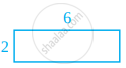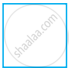(a) The area of the rectangle is ______.

(b) The area of the square is ______.

Exercise | Q 13.(a) | Page 96

1 m = ______ cm.

Exercise | Q 13.(b) | Page 96

1 sq cm = ______ cm × 1 cm.

Exercise | Q 13.(c) | Page 96

1 sq m = 1 m × ______ m = 100 cm × ______ cm.

Exercise | Q 13.(d) | Page 96

1 sq m = ______ sq cm.

#### State whether the following statement is True or False:

Exercise | Q 14 | Page 96

If length of a rectangle is halved and breadth is doubled then the area of the rectangle obtained remains same.

• True

• False

Exercise | Q 15 | Page 96

Area of a square is doubled if the side of the square is doubled.

• True

• False

Exercise | Q 16 | Page 96

Perimeter of a regular octagon of side 6 cm is 36 cm.

• True

• False

Exercise | Q 17 | Page 96

A farmer who wants to fence his field, must find the perimeter of the field.

• True

• False

Exercise | Q 18 | Page 96

An engineer who plans to build a compound wall on all sides of a house must find the area of the compound.

• True

• False

Exercise | Q 19 | Page 96

To find the cost of painting a wall we need to find the perimeter of the wall.

• True

• False

Exercise | Q 20 | Page 96

To find the cost of a frame of a picture, we need to find the perimeter of the picture.

• True

• False

Exercise | Q 21 | Page 96

Four regular hexagons are drawn so as to form the design as shown in the given figure. If the perimeter of the design is 28 cm, find the length of each side of the hexagon.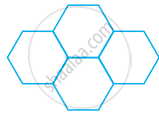Exercise | Q 22 | Page 96

Perimeter of an isosceles triangle is 50 cm. If one of the two equal sides is 18 cm, find the third side.

Exercise | Q 23 | Page 96

Length of a rectangle is three times its breadth. Perimeter of the rectangle is 40 cm. Find its length and width.

Exercise | Q 24 | Page 96

There is a rectangular lawn 10m long and 4m wide in front of Meena’s house (see the figure). It is fenced along the two smaller sides and one longer side leaving a gap of 1m for the entrance. Find the length of fencing.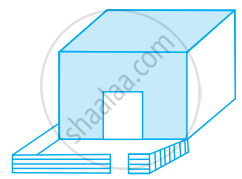Exercise | Q 25 | Page 97

The region given in the following figure is measured by taking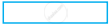as a unit. What is the area of the region?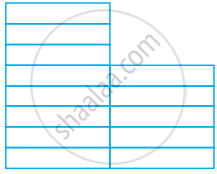Exercise | Q 26 | Page 97

Tahir measured the distance around a square field as 200 rods (lathi). Later he found that the length of this rod was 140 cm. Find the side of this field in metres.

Exercise | Q 27 | Page 97

The length of a rectangular field is twice its breadth. Jamal jogged around it four times and covered a distance of 6 km. What is the length of the field?

Exercise | Q 28 | Page 97

Three squares are joined together as shown in figure. Their sides are 4 cm, 10 cm and 3 cm. Find the perimeter of the figure.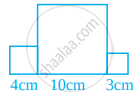Exercise | Q 29 | Page 97

In the given figure, all triangles are equilateral and AB = 8 units. Other triangles have been formed by taking the mid points of the sides. What is the perimeter of the figure?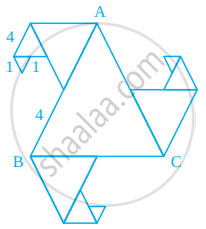Exercise | Q 30 | Page 97

Length of a rectangular field is 250 m and width is 150 m. Anuradha runs around this field 3 times. How far did she run? How many times she should run around the field to cover a distance of 4 km?

Exercise | Q 31 | Page 97

Bajinder runs ten times around a square track and covers 4 km. Find the length of the track.

Exercise | Q 32 | Page 98

The lawn in front of Molly’s house is 12 m × 8 m, whereas the lawn in front of Dolly’s house is 15 m × 5 m. A bamboo fencing is built around both the lawns. How much fencing is required for both?

Exercise | Q 33 | Page 98

The perimeter of a regular pentagon is 1540 cm. How long is its each side?

Exercise | Q 34 | Page 98

The perimeter of a triangle is 28 cm. One of it’s sides is 8cm. Write all the sides of the possible isosceles triangles with these measurements.

Exercise | Q 35 | Page 98

The length of an aluminium strip is 40 cm. If the lengths in cm are measured in natural numbers, write the measurement of all the possible rectangular frames which can be made out of it. (For example, a rectangular frame with 15 cm length and 5 cm breadth can be made from this strip.)

Exercise | Q 36 | Page 98

Base of a tent is a regular hexagon of perimeter 60 cm. What is the length of each side of the base?

Exercise | Q 37 | Page 98

In an exhibition hall, there are 24 display boards each of length 1m 50 cm and breadth 1 m. There is a 100m long aluminium strip, which is used to frame these boards. How many boards will be framed using this strip? Find also the length of the aluminium strip required for the remaining boards.

Exercise | Q 38 | Page 98

In the above question, how many square metres of cloth is required to cover all the display boards? What will be the length in m of the cloth used, if its breadth is 120 cm?

Exercise | Q 39 | Page 98

What is the length of outer boundary of the park shown in the given figure? What will be the total cost of fencing it at the rate of Rs 20 per metre? There is a rectangular flower bed in the center of the park. Find the cost of manuring the flower bed at the rate of Rs 50 per square metre.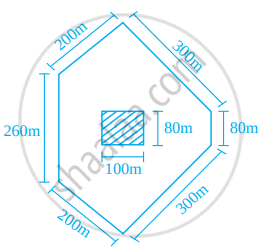Exercise | Q 40 | Page 99

Total cost of fencing the park shown in the given figure is Rs 55000. Find the cost of fencing per metre.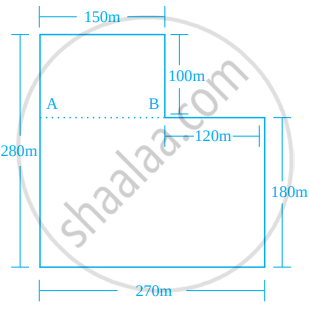Exercise | Q 41 | Page 99

In the given figure, each square is of unit length
(a) What is the perimeter of the rectangle ABCD?
(b) What is the area of the rectangle ABCD?
(c) Divide this rectangle into ten parts of equal area by shading squares. (Two parts of equal area are shown here)
(d) Find the perimeter of each part which you have divided. Are they all equal?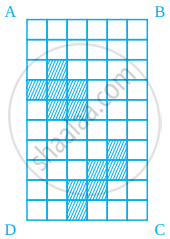Exercise | Q 42 | Page 99

Rectangular wall MNOP of a kitchen is covered with square tiles of 15cm length (see figure)). Find the area of the wall.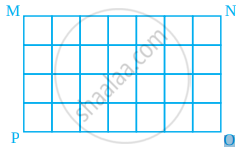Exercise | Q 43 | Page 100

Length of a rectangular field is 6 times its breadth. If the length of the field is 120 cm, find the breadth and perimeter of the field.

Exercise | Q 44 | Page 100

Anmol has a chart paper of measure 90 cm × 40 cm, whereas Abhishek has one which measures 50 cm × 70 cm. Which will cover more area on the table and by how much?

Exercise | Q 45 | Page 100

A rectangular path of 60 m length and 3 m width is covered by square tiles of side 25 cm. How many tiles will there be in one row along its width? How many such rows will be there? Find the number of tiles used to make this path?

Exercise | Q 46 | Page 100

How many square slabs each with side 90cm are needed to cover a floor of area 81 sq m.

Exercise | Q 47 | Page 100

The length of a rectangular field is 8 m and breadth is 2 m. If a square field has the same perimeter as this rectangular field, find which field has the greater area.

Exercise | Q 48 | Page 100

Parmindar walks around a square park once and covers 800 m. What will be the area of this park?

Exercise | Q 49 | Page 100

The side of a square is 5 cm. How many times does the area increase, if the side of the square is doubled?

Exercise | Q 50 | Page 100

Amita wants to make rectangular cards measuring 8 cm × 5 cm. She has a square chart paper of side 60 cm. How many complete cards can she make from this chart? What area of the chart paper will be left?

Exercise | Q 51 | Page 100

A magazine charges Rs 300 per 10 sq cm area for advertising. A company decided to order a half page advertisment. If each page of the magazine is 15 cm × 24 cm, what amount will the company has to pay for it?

Exercise | Q 52 | Page 100

The perimeter of a square garden is 48 m. A small flower bed covers 18 sq m area inside this garden. What is the area of the garden that is not covered by the flower bed? What fractional part of the garden is covered by flower bed? Find the ratio of the area covered by the flower bed and the remaining area.

Exercise | Q 53 | Page 100

Perimeter of a square and a rectangle is same. If a side of the square is 15 cm and one side of the rectangle is 18 cm, find the area of the rectangle.

Exercise | Q 54 | Page 101

A wire is cut into several small pieces. Each of the small pieces is bent into a square of side 2 cm. If the total area of the small squares is 28 square cm, what was the original length of the wire?

Exercise | Q 55 | Page 101

Divide the park shown in the figure of question 40 into two rectangles. Find the total area of this park. If one packet of fertilizer is used for 300 sq m, how many packets of fertilizer are required for the whole park?

Exercise | Q 56 | Page 101

The area of a rectangular field is 1600 sq m. If the length of the field is 80 m, find the perimeter of the field.

Exercise | Q 57 | Page 101

The area of each square on a chess board is 4sqcm. Find the area of the board.
(a) At the beginning of game when all the chess men are put on the board, write area of the squares left unoccupied.
(b) Find the area of the squares occupied by chess men.

Exercise | Q 58.(a) | Page 101

Find all the possible dimensions (in natural numbers) of a rectangle with a perimeter 36 cm and find their areas.

Exercise | Q 58.(b) | Page 101

Find all the possible dimensions (in natural numbers) of a rectangle with an area of 36 sq cm, and find their perimeters.

Exercise | Q 59.(i) | Page 101

Find the area and Perimeter of the following figure, if area of small square is 1 sq cm.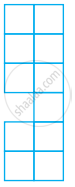Exercise | Q 59.(ii) | Page 101

Find the area and perimeter of the following figure, if area of small square is 1 sq cm.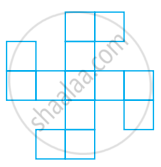Exercise | Q 59.(iii) | Page 101

Find the area and perimeter of the following figure, if area of small square is 1 sq cm.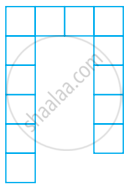Exercise | Q 60 | Page 102

What is the area of each small square in the given figure. if the area of entire figure is 96 sq cm. Find the perimeter of the figure.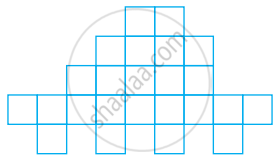## Chapter 6: Mensuration

Exercise## NCERT solutions for Mathematics Exemplar Class 6 chapter 6 - Mensuration

NCERT solutions for Mathematics Exemplar Class 6 chapter 6 (Mensuration) include all questions with solution and detail explanation. This will clear students doubts about any question and improve application skills while preparing for board exams. The detailed, step-by-step solutions will help you understand the concepts better and clear your confusions, if any. Shaalaa.com has the CBSE Mathematics Exemplar Class 6 solutions in a manner that help students grasp basic concepts better and faster.

Further, we at Shaalaa.com provide such solutions so that students can prepare for written exams. NCERT textbook solutions can be a core help for self-study and acts as a perfect self-help guidance for students.

Concepts covered in Mathematics Exemplar Class 6 chapter 6 Mensuration are Mensuration, Concept of Perimeter, Perimeter of a Rectangle, Perimeter of Squares, Perimeter of Triangles, Perimeter of Polygon, Concept of Area, Area of Rectangle, Area of Square.

Using NCERT Class 6 solutions Mensuration exercise by students are an easy way to prepare for the exams, as they involve solutions arranged chapter-wise also page wise. The questions involved in NCERT Solutions are important questions that can be asked in the final exam. Maximum students of CBSE Class 6 prefer NCERT Textbook Solutions to score more in exam.

Get the free view of chapter 6 Mensuration Class 6 extra questions for Mathematics Exemplar Class 6 and can use Shaalaa.com to keep it handy for your exam preparation